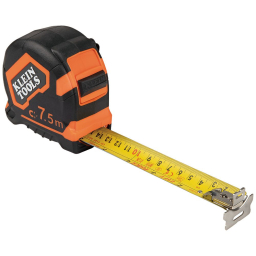# Issac 2

Issac bought some wire for his project. He used 1/3 of the wire and had 80 cm of wire left. How much wire did he have at first

x =  120 cm

### Step-by-step explanation:Did you find an error or inaccuracy? Feel free to write us. Thank you!

Tips for related online calculators
Need help calculating sum, simplifying, or multiplying fractions? Try our fraction calculator.
Do you have a linear equation or system of equations and looking for its solution? Or do you have a quadratic equation?